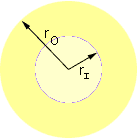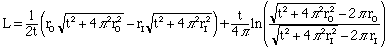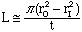Subject: Varying Diameters for Linear Feet Name: Richard Who is asking: Other Level: All Question: Although I have been able to solve my equation using an Excel spreadsheet, I have yet to duplicate it in a written constant formula. Sounds odd I know, however I am very curious and would like very much to do this. I would appreciate any help. The root of my equation is simply this, I have a roll of paper, wrapped around a corrugate core, whos diameter is 10.750 in. The outer diameter of the roll is approx. 60 in. The thickness of the paper is .014 in. I am trying to find out how much linear feet of paper is left on the roll, given only the diameter of paper remaining on the core, I'll call the remaining paper scrap. For example, if I had a diameter of 10.750, there would be no paper on the core. If I had a diameter of 60.002 in. my scrap would be 16297.392 ft. I believe this is correct because I used a spreadsheet to get the answer. Because of the ever changing diameter of the roll as it is being consumed, is there a formula that I can use in a small Visual Basic program to identify the ramaining footage (scrap) based on 2 inputs, the thickness of the paper and the diameter of the roll. Thank you Richard Hi Richard, The paper is rolled around the core in the form of a spiral called the spiral of Archimedes. Using some calculus you can find an expression for the length of the spiral.If rO is the radius of the outside of the roll of paper and rI is the radius of the core as in the diagram and t is the thickness of the paper then the length of the scrap is given bywhere ln is the natural logarithm. If you perform this calculation with the data given in your problem, rO = 60.002/2 = 30.001 inches, rI = 10.750/2 = 5.375 inches and t = 0.014 inches then L = 195490 inches or 16290.8 feet. If t is much smaller than rO and rI, which it is in your situation, there is an approximation of L which is much easier to calculate. In this situationIn the example above this also gives L = 195490 inches = 16290.8 feet. Cheers, Chris and Harley In August of 2006 we received the following from Marcia about the use of this formula in her work. At Chapman Data Services we laser personalize direct mail campaigns. Our client may buy 1 million forms that arrive on rolls of approximately 30,000 forms per roll. They use the forms up over the course of several months to mail several different projects of varying quantities. We needed a formula in order to do inventory on the partial rolls that are kept for future mailings. There is waste involved each time we start and stop so it is difficult to track usage just by subtracting mail quantities from received quantities. We considered doing it by weight but a scale is expensive. We are currently testing the formula to tweak the paper thickness and expect it to work well. Marcia

Go to Math Central